• 검색 결과가 없습니다.

# OXIDATION OF MICROCYSTINS BY PERMANGANATE, OZONE AND FERRYL-TETRAPOLYPHOSPHATE COMPLEX: KINETICS AND MECHANISM

N/A
N/A
Protected

Share "OXIDATION OF MICROCYSTINS BY PERMANGANATE, OZONE AND FERRYL-TETRAPOLYPHOSPHATE COMPLEX: KINETICS AND MECHANISM"

Copied!
135
0
0
더 보기 ( 페이지)

전체 글

## Introduction

Research bacground

• Environmental issues of microcystins
• MCs in drinking water treatment plant
• Chemical oxidation of MCs

### Limitations of the previous works

• Overview
• Oxidation of MCs by Mn(VII)
• Oxidation of MCs by O 3
• Oxidation of MCs by Fe(IV)-TPP complex

Despite the above-mentioned studies, some important topics about the oxidation of MC by Mn(VII) remain unknown and partially misinterpreted. Despite the above-mentioned studies, several important topics on the oxidation of MC by O3 ... remain unknown and partially unclear.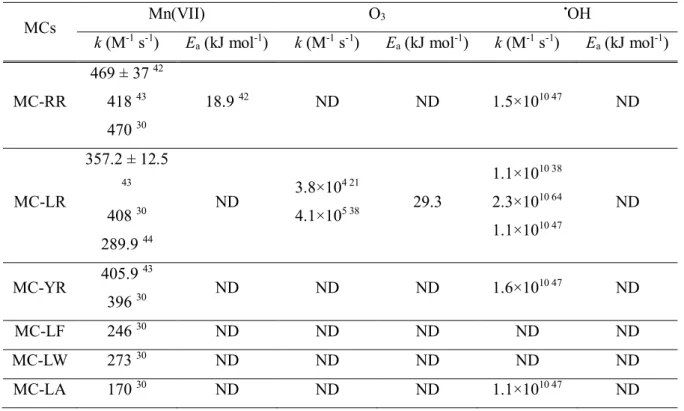Table 1.2. Summary of kinetics for the reactions of six MCs with Mn(VII), O 3 and • OH

### Objectives of the study

Analytical methods. Measurements of MCs, oxidation products of MC-LR, HCHO, Fe(II), H2O2 were the same as that of section 2.2.4. Effects of TPP:Fe(II) molar ratio and H2O2 concentration on the degradation of MC-LR by the Fe(II)-TPP/H2O2 system. The oxidation of MC-LR by the Fe(II)-TPP/H2O2 system was tested by varying [TPP]/[Fe(II)] and H2O2 (refer to Table 2.4 and Figure 3.51b).

## Materials and Methods

### Oxidation of MCs by Mn(VII)

• Reagents
• Determination of rate constants
• Natural water samples
• Analysis of MCs and oxidation products of MC-LR
• Analysis of Mn(VII) in natural waters
• DOM characterization
• Computational pK a prediction for MCs

No pH buffers were used for experiments at pH 2–4; the pH changes during the reactions were less than 0.2 units. The example of the measured absorbance and the calculated Mn(VII) during the reaction of Mn(VII) in Maegok water is shown in Figure 2.3.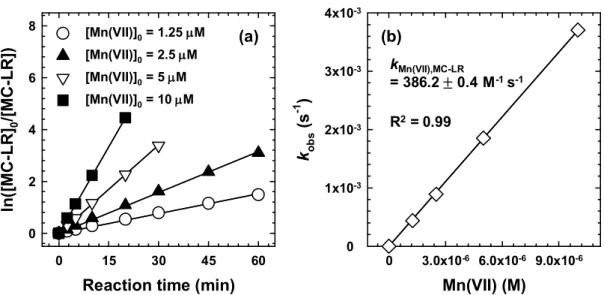Figure 2.1. Example of the determination of k Mn(II),MC . (a) Time-dependent profiles of ln([MC- ln([MC-LR]) 0 /[MC-LR]) at different Mn(VII) doses, and (b) plot of k obs as a function of Mn(VII) concentration ([MC-LR] 0 = 0.1 μM, [Mn(VII)] 0 =

### Oxidation of MCs by O 3

• Reagents
• Determination of rate constants
• Natural water samples
• Analysis of O 3 in natural waters
• Analytical methods

After ozonation, the residual concentrations of target MCs and CAs were determined by RSLC. The red and dashed lines represent absorbance adjustments with respect to the entire investigated reaction time or stationary phases (from 2 to 3 s).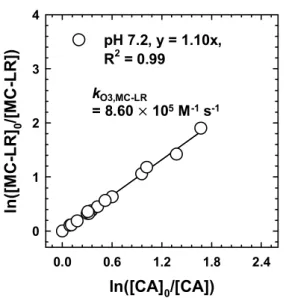Figure 2.5. Example of the determination of k O3,MC . CK plot of ln([MC-LR] 0 /[MC-LR]) versus ln([CA] 0 /[CA]) at different O 3 doses ([MC-LR] 0 = 0.1 μM, [CA] 0 = 0.1 μM, [O 3 ] 0 = 0.02-0.2 μM, [t-BuOH] 0 = 5 mM, temperature = 20 ± 1°C, pH =

### Oxidation of MC-LR by Fe(IV)-TPP complex

• Reagents
• Experimental procedure
• Experimental design for RSM analysis
• Analytical methods

Effects of various water quality parameters on the oxidation of MC-LR by Mn(VII) ([MC-. The chromatogram at 0 min with mass spectra (left) and time-dependent chromatograms (right) of MC-LR. Based on some of the identified oxidation products , it was demonstrated that the oxidation of MC-LR by Mn(VII) generally proceeded in the direction of destruction of the Adda unit (which is the functional group responsible for the hepatotoxicity of MCs).

Oxidative degradation of MC-LR by Fe(II)/O2 and Fe(II)/H2O2 systems was examined in the absence and presence of iron-chelating agents (ligand: EDTA, oxalate and TPP) at neutral pH (Figure 3.50). The oxidation of MC-LR by Fe(II)-TPP/O2 and Fe(II)-TPP/H2O2 systems was strongly dependent on pH.

## Results and Discussion

### Kinetics for the reactions of MCs with Mn(VII)

First, the direct reactions of those amino acids in the X and Y positions with Mn(VII) can be considered. Second, the amino acids in the X and Y positions can change the electron density of reaction sites; alkene groups in the Adda and Mdha units of MC structure are known to be the primary oxidation sites by Mn(VII) (refer to Figure It appears that electron donating and - withdrawing properties of the amino acids in the X and Y positions the π-d electron density of the alkenes through electronic (inductive/resonance) effects.108, 109 This explanation is quite interesting, because the alkene groups are relatively far (four to six bonds away) from the amino acids in the X and Y positions (refer to Figure 1.3 ) Electronic effect of the amino acids located in the X and Y positions in MC structure on the reactivities of alkene groups with Mn(VII).

Relatively low ∆H‡and high negative ∆S‡values ​​were also reported for reactions of other alkene compounds with Mn(VII) (Table On the basis of the determined Eavalues, the kMn(VII),MC values ​​vary with M-1s- 1 depending on the MC in the temperature range of 10.5–37.0°C, where the bloom of Microcystis aeruginosa generally occurs.115, 116 The removal efficiency of MCs with Mn(VII) may change with temperature accordingly. For all MC species-specific rate constants were in the order kMn(VII),MC1>kMn(VII),MC2>kMn(VII),MC3 » kMn(VII),MC4, except for MC-YR, which showed high kMn(VII ),MC4 and kMn(VII),MC5 values.

### Oxidative degradation of MCs in natural waters

The oxidative degradation of MCs by Mn(VII) was investigated in five natural water samples spiked with MCs at trace concentrations (10 nM).Figure 3.9. Absorbance spectra during the decomposition of Mn(VII) in natural waters. The lightest lines are the final spectra ([Mn(VII)] 0 = 2.5 μM, temperature = 21 ± 1°C).

### Effects of DOM characteristics on ò[Mn(VII)]dt

However, the type and concentration of DOM strongly influenced the oxidation of MC-LR by Mn(VII) (Figure 3.11d-f), which is consistent with the results of previous studies.27, 44 This inhibitory effect of DOM fractions was in the order SRHA > SRFA > NaHA. A total of nine DOM characteristics of natural waters were correlated with ò[Mn(VII)]dt (Figure 3.14). Using the calculated ò[Mn(VII)]dt values ​​and Equation 3.2, the removal efficiencies of the six MCs in the five natural waters were modeled and the results compared with the experimental data (Figure 3.15).

Using the calculated ∫[Mn(VII)]dt values ​​and equation 3.2, the removal efficiency of MC-LR in the three natural waters was modeled and the results were compared with the experimental data. Lines represent the obtained linear regression model (solid) and the 95% prediction interval (dashed line) from the data in Figure 3.14b, c and i.

### Oxidation products and pathways

Two previous studies have proposed the oxidation mechanism of MC-LR by Mn(VII) based on the product study by LC/MS.28, 44 But a majority. The oxidation pathways of MC-LR with Mn(VII) were proposed based on the identified products (Figure 3.37). There are three sites that Mn(VII) is thought to attack in the MC-LR structure (sites A–C): two alkene groups in Adda (sites A and B) and one alkene group in Mdha (site C) (see Figure 3.37 ).

The alkene oxidation by Mn(VII) under the neutral pH conditions begins with the formation of the cyclic hypomanganate(V) ester as an intermediate (denoted as Ia). All reaction pathways in the process of MC-LR oxidation by Mn(VII) (Figure 3.37) can be successfully explained by the transformation of sites A–C via Reactions I–V.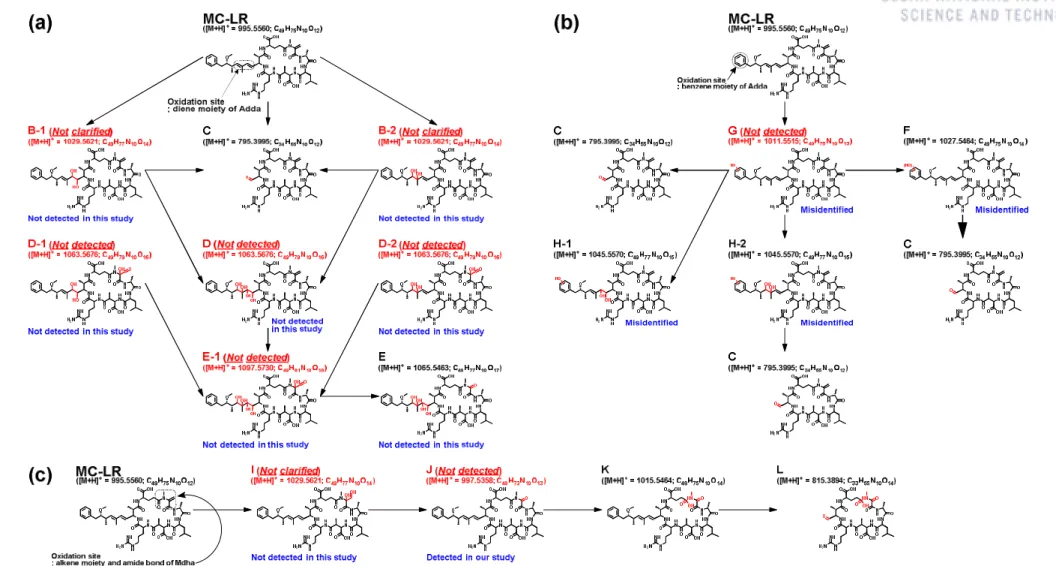Figure 3.17. Oxidation pathways of MC-LR by Mn(VII) proposed by Jeong et al. 44 : (a) diene oxidation products, (b) aromatic ring oxidation products, and (c) amide bond hydrolysis products

### Kinetics for the reactions of MCs with O 3

Kinetics for the reactions of MCs with O3. To assess the reactivity of the six selected MCs with O3, kO3,MC values ​​were determined using CK with CA as competitor at different pH values ​​(pH 6.2-9 at 20. The kO3,CA values ​​were calculated from the slope of the linear plot of the pseudo-first order rate constant (kobs) versus initial O3 concentrations using SFS at pH 2.3-9 and 7.5-25°C (Figure 3.41). All kO3, MC values ​​expected for kO3,MC-LW show similar values ​​to kO3,CA (see the slopes in Figures 3.39b-f), indicating that the dominant oxidation mechanism of MCs by O3 is alkene oxidation.

The values ​​for the reactions of MCs with O3 were calculated to be 22.6-31.9 kJ mol-1 from the slope of the Arrhenius plot (Figure 3.43b). Kinetics for the reactions of MCs with ·OH. To evaluate the reactivity of six selected MCs with ·OH, k·OH, MC values ​​were determined using CK with pCBA as competitor at different pH (pH 6.2‒.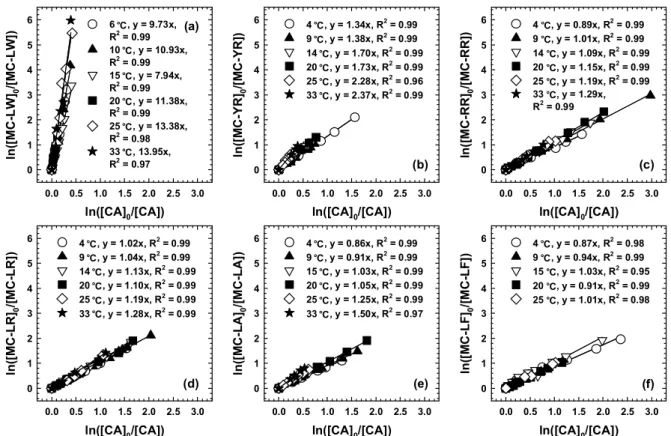Figure 3.40. Variations of ln([MC] 0 /[MC]) as a function of corresponding ln([CA] 0 /[CA]) during the ozonation at temperatures ([MC] 0 = 0.1 μM, [CA] 0 = 0.1 μM, [O 3 ] 0 = 0.025–0.25 μM, [t-BuOH] 0 = 5 mM, pH = 7.2).

### Kinetics for the reactions of MCs with · OH

Although the original report, which for the first time investigated the rate constants for the reaction of various aromatic compounds with ·OH, gave the k·OH,pCBAas 5.0 x 109 M-1s-1 at pH 6-9.4, no information is given about the reaction temperature or the deEa for the reaction of pCBA with ·OH (Ea, ·OH,pCBA). Therefore, in this study, the Ea,·OH,pCBA value was determined using the CK method with HCHO as competitor of which the second-order constant for HCHO with ·OH (k·OH,HCHO) and its temperature dependence were estimated to be 3, 9. × 108M-1 s-1 at 25°C and 13 kJ mol-1, respectively. The estimated Ea values ​​for the reaction of MCs with ·OH values ​​were also very similar to each other, ranging from 35.1 to 36.7 kJ mol-1 (Figure 3.47b).

These similar reactivities of MCs toward ·OH can be elucidated by the highly reactive and nonselective nature of ·OH. To verify the validity of the determined kO3,MC and k·OH,MC values, the oxidative degradation of MCs by different O3 doses was investigated in five cases.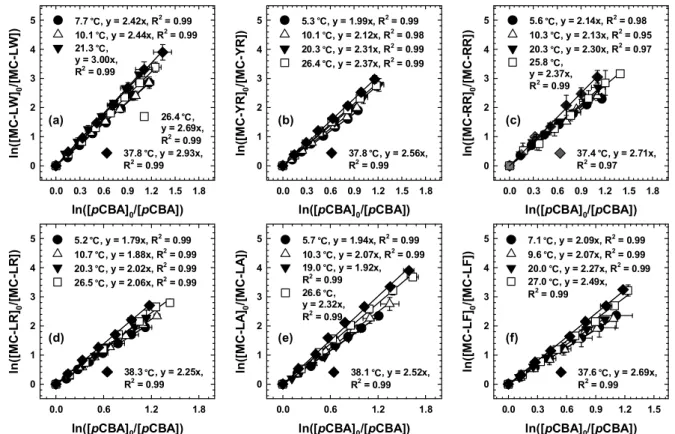Figure 3.45. Variations of ln([MC] 0 /[MC]) as a function of corresponding ln([pCBA] 0 /[pCBA]) during the oxidation reaction in UV/H 2 O 2 system at different temperatures ([MC] 0 = 0.1 μM, [CA] 0 = 0.1 μM, [H 2 O 2 ] 0 = 1 mM, temperature = 20 ± 1°C).

MC-LR degradation by the Fe(II)/O2 and Fe(II)/H2O2 systems in the presence of ligands. The degradation of MC-LR in the absence of ligands was minor for both the Fe(II)/O2 and Fe(II)/H2O2 systems. The Fe(II) ligand/O2 and Fe(II) ligand/H2O2 systems showed different patterns in terms of the kinetics of MC-LR degradation.

Degradation of MC-LR by Fe(II)-ligand/O2 systems is mainly attributed to oxidation by Fe(IV) species produced via Reactions. The faster degradation of MC-LR from the Fe(II)-ligand/H2O2 system compared to the Fe(II)-ligand/O2 systems (Figure 3.50) suggests that Reaction 3.6 is faster than Reaction 3.4 in the presence of ligands.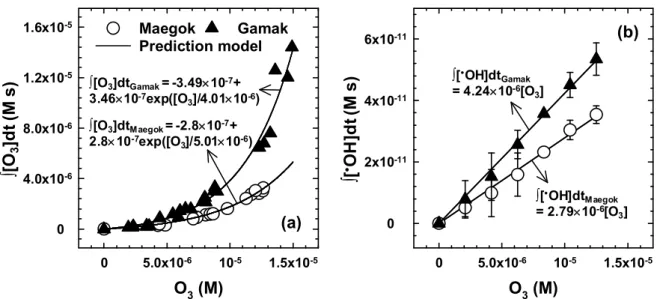Figure 3.49. ∫[O 3 ]dt and (b) ∫[ • OH]dt as a function of O 3 concentration. Symbols and lines represent experimental data and prediction model, respectively.

ANOVA for regression model of MC-LR degradation efficiency (Y1) as a function of Fe(II) (X1) and TPP (X2) concentrations (for Figure 3.52a). Plot of residual normal probability for the MC-LR degradation efficiency model from (a) Fe(II)-TPP/O2 and (b) Fe(II)-TPP/O2 system. Increasing the concentration of Fe(II) increases the production of oxidants, thereby increasing the degradation of MC-LR.

The degradation efficiencies of MC-LR (after 10 min) were fitted to the model equation and the response surface was plotted (Figure 3.52b). The degradation of MC-LR was examined by changing the pH from 3 to 11 in Fe(II)-TPP/O2 and Fe(II)-TPP/H2O2 systems.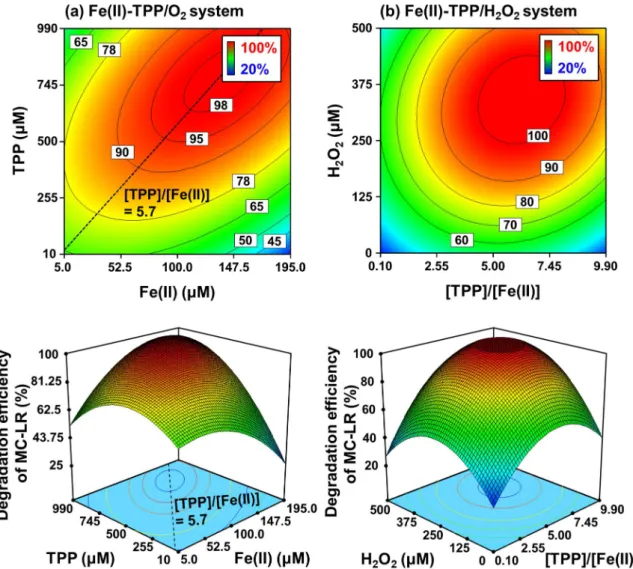Figure 3.52. Effects of Fe(II), TPP, and H 2 O 2 doses on the degradation efficiency of MC-LR by Fe(II)- Fe(II)-TPP/O 2 (a) and Fe(II)-TPP/H 2 O 2 systems ([MC-LR] 0 = 0.201 mg/L (0.2 μM), pH = 7, [Fe(II)] 0 = 100 μM for (b), reaction time = 60 min f

### Effect of pH

Therefore, the pH-dependent MC-LR oxidation by the Fe(II)-TPP/H2O2 system is solely the result of the speciation of Fe(IV)-TPP complexes with different reactivities. Based on the above statements regarding the Fe(IV)-TPP speciation, it is postulated that the Fe(IV)-TPP species produced at pH 7 (where the MC-LR oxidation is maximized in both the Fe(II) )-TPP/O2 and Fe(II)-TPP/H2O2 systems) has a higher reactivity than the species produced at pH 8 (minimizing the degradation of MC-LR in both systems). Because an oxidant with high reactivity generally exhibits poor selectivity, the Fe(IV)-TPP species at pH 7 should be less selective than those at pH 8.

The results in Figure 3.56 clearly show that the difference in the degradation efficiency of different MCs was much less at pH 7 (3.48%) than at pH 7, proving that the oxidant produced at pH 7 is less selective than that at pH 8 (refer also to the time-concentration profiles of the six MCs during their oxidation, Figure 3.57). At pH 8, the degradation efficiency of MCs was in the order of MC-YR > MC-LW > MC-LF >.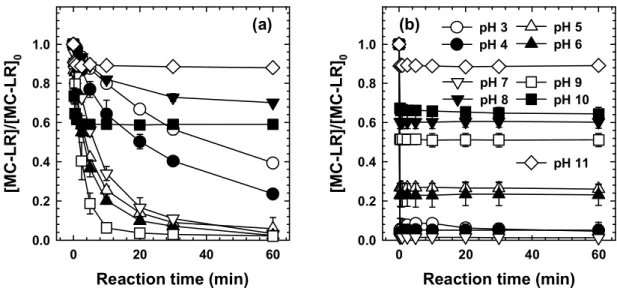Figure 3.55. Degradation of MC-LR by Fe(II)-TPP/O 2 (a) and Fe(II)-TPP/H 2 O 2 (b) systems at different pH values ([MC-LR] 0 = 0.201 mg/L (0.2 μM); [Fe(II)] 0 = 100 μM; [TPP] 0 = 500 μM; [H 2 O 2 ] 0 = 250 μM).

### Oxidation products and pathways

To gain an insight into the oxidation mechanism of MC-LR by the Fe(IV)-TPP complex, the oxidation products formed during MC-LR degradation of. The degradation pathways of MC-LR were postulated along the four sites and the identified oxidation products (Figure 3.82). These hydroxylated products have also been observed after oxidation of MC-LR by SO4•-,59 TiO2 photocatalysis,151 and ferrate135.

To verify whether ·OH can induce MC-LR oxidation via a route analogous to that proposed in Figure 3.84, the UV/H2O2 system was applied to oxidize MC-LR, for monitoring the oxidation products. For example, HCHO production during the degradation of MC-LR was compared in the Fe(II)-TPP/O2 and UV/H2O2 systems; the time concentration profiles of HCHO are shown in Figures 3.89 and 3.90.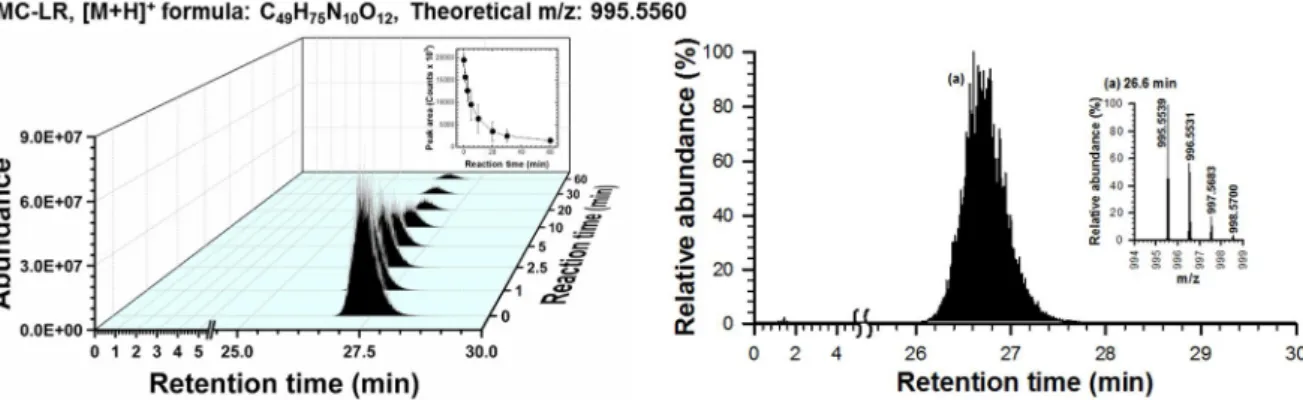Figure 3.58. LC/MS identification of MC-LR ([M+H] + = 995.5560). The chromatogram at 20 min with mass spectra (left) and time-dependent chromatograms of MC-LR (right).

### Hepatotoxicity test

Oxidation of the cyanobacterial hepatotoxin microcystin-LR by chlorine dioxide: reaction kinetics, characterization and toxicity of the reaction products. Oxidation of the cyanobacterial hepatotoxin microcystin-LR by chlorine dioxide: Effect of natural organic matter. Mechanistic studies of the photocatalytic oxidation of microcystin-LR: an investigation of the by-products of the degradation process.

Oxidation of microcystin-LR by the Fenton process: Kinetics, degradation intermediates, water quality and toxicity assessment. Oxidation of microcystin-LR by copper(II) coupled with ascorbic acid: Kinetics modeling towards formation of H2O2.

Conclusions

수치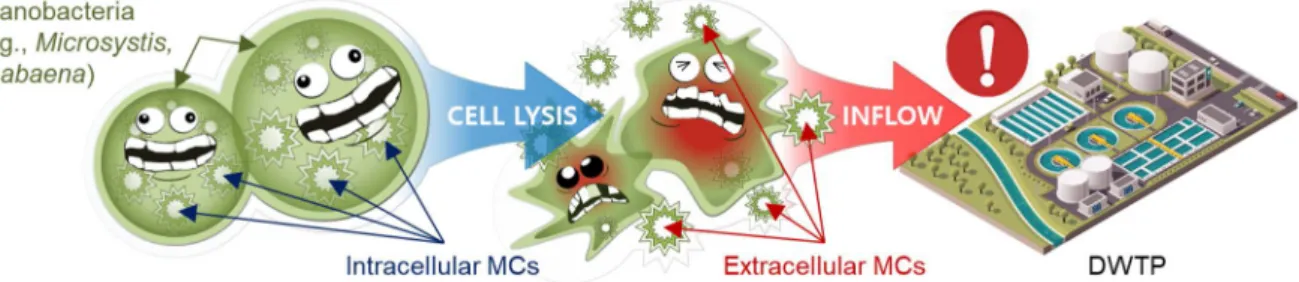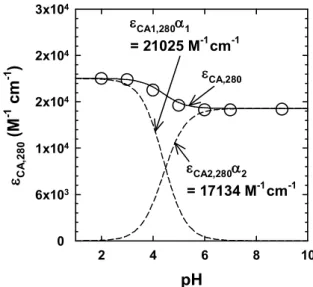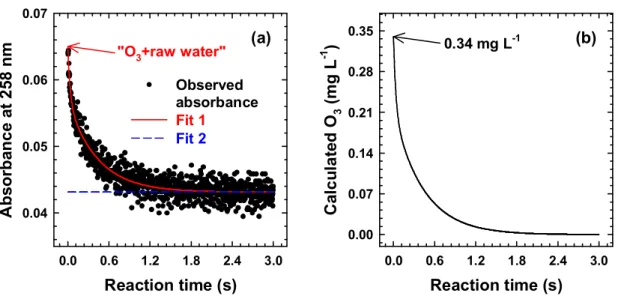+7

참조

관련 문서

MRS is to measure very small metabolite signals, whose resonant frequencies spread over the chemical shift range characteristic of the measured nucleus.

In the results, VO 2 max has significantly increased according to the degree of the treadmill inclination, and M4 group (larger body type) consumed more oxygen than the

Correlated Functions of Dual-Channel Differential Signals Quadrature (Sine and Cosine) Signals such as PECL, LVPECL and LVDS digital signals or automotive sensors

generalization of the exponential integral and to further derive some inequalities for the generalized function2. The results are presented in

Correlating with the results from the above characterization, it is found that the main causes for the water deactivation of the Cu/Zn catalyst were the water occlusion

Of the domestic homicides recorded in the NHMP in 2010–12, the majority were classified as an intimate partner homicide. The frequency of intimate partner homicides has

22 By rearranging the terms, the equation can be shown to identify the level of capital in the foreign jurisdiction where the tax advantage of locating the subsequent unit

ration activity changes di¤erentially with respect to pass-through business activity in states that change either corporate or personal tax rates, an approach related to Yagan

Activities that make people aware of their travel options, encourage them to try new modes and give them the skills they need to use them are being timed and geographically

언어 선택하기

웹사이트는 선택한 언어로 번역됩니다.

기타 언어